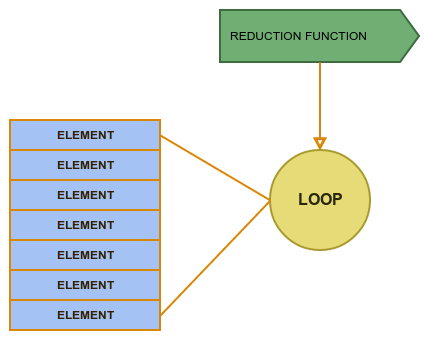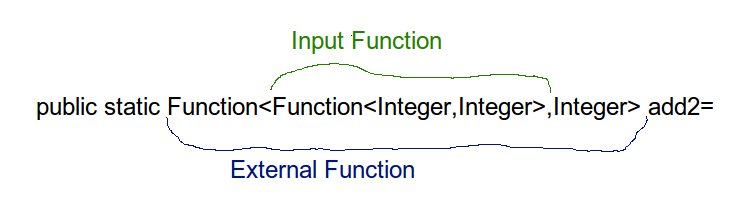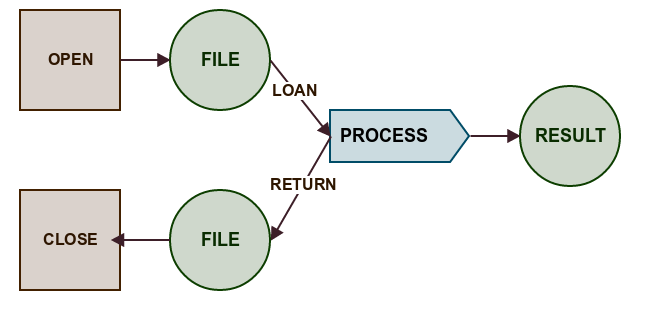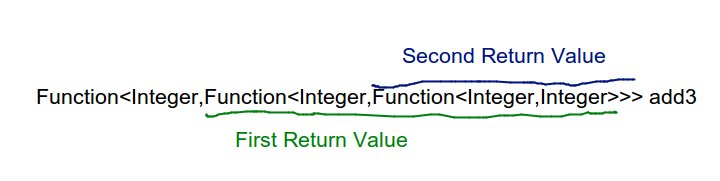# Functions in Java - Intro

This workshop is an introduction to Functions and Functional Programming in Java8. We are going to learn the new code syntax but also we are going to practice new programming concepts and new ways of solving problems with Functional mechanisms added to Java 8 .

• In the first part we will learn how to use new syntax to define functions. How to create a function and how to compose multiple functions together.
• In the second part we will understand concept of a function as a value and how passing a function to method can help avoid repeatition in code.
• In the next part finally we are going to walk through plenty of new functional intefaces like BiFunction,Consumer or Prediicate which were added to Java 8.
• Part four is about "function as a result value" - we will see how to return a function from a method , how to do dependency injection in Functional Style and what is "Currying"
• Next part will be about the real world and how to use pure functions when we need to deal with exceptions and missing values.
• We will finish by looking at some theoretical examples to gain broader understanding of a concept of encapsualtion and why Referential Transparency is crucial in Functional Programming.

# Part 1 : Define a FunctionDeclaring simple function should be simple and that's why it is enough to write following one line to define parsing String function :

`````` Function<String,Integer> parseString= s1->Integer.parseInt(s1);
``````

However in versions before Java8 we would have to write full definition of an anonymous class :

``````Function<String,Integer> parseStringLong=new Function<String, Integer>() {
@Override
public Integer apply(String s) {
return Integer.parseInt(s);
}
};
``````

We will analyze step by step how exactly the second form is shortened to the first form.

## Composition

When you have two independent functions and result of the first one has the same type as the input of the second one then we can easily compose them into single function :

f:A=>B , g:B=>C f andThen g : A=>C

`````` Function<Integer,Integer> f= i -> i+1
Function<String,Integer> parseString= s1->Integer.parseInt(s1);
Function<String, Integer> parseStringAddOne = parseString.andThen(f);
``````

This way we can encapsulate usage of each smaller function under "composed facade".

## Tuples

This is a very convenient structure which just represent "two or more values tied together" - so we don't need to define project specific pair class. In Java we can use library Javaslang which defines family Tuple classes.

``````Tuple2<String, Integer> t2 = Tuple.of("value", 2);
``````

Tuples provide a lot of useful transformations which receive functions as an argument - we will see this in the next part.

## Closures and Effectively Final

Finally we can use external values in the functions but there is one rule - those values must not be changed (the definition of effectivelly final)!

``````double taxRate=0.23;
//must not change taxRate value!
Function<Integer,Double> gross=net -> net+(taxRate*net);
``````

## Exercises

Level 1

Define and compose simple functions

Level 2

Write custom andThen and compose (without using Function.andThen or Function.compose)Level 3

Write andThen with Generics (without using Function.andThen or Function.compose)

# Part 2 : Function as a value

We will see how treating function as a value help us a lot to abstract part of functionality and create more general constructs (a.k.a DRY):

For example :

We have two very similar methods :

``````private Integer sum(final Integer init,final Collection<Integer> c){
Integer result=init; // encapsulation? why parameters are final - wait for part 6
for (Integer i : c) {
result = result+i;
}
return result;
}

private Integer multiply(final Integer init,final Collection<Integer> c){
Integer result=init; // encapsulation? why parameters are final - wait for part 6
for (Integer i : c) {
result = result*i;
}
return result;
}
``````

Which differs by only one operation so we can move this operation to a function which can be a parameter in more general function (so more general function is parameterized not only by values but also by behaviour) :

``````   private Integer reduce(Integer init, final Collection<Integer> c,BiFunction<Integer,Integer,Integer> f){
Integer result=init;
for (Integer i : c) {
result = f.apply(result,i);
}
return result;
}
``````

And now we can very easily define sum in terms of reduce

``````Integer sumReduce = reduce(0, asList(1, 2, 3, 4, 5),(a,b)->a+b);
Integer multiplyReduce = reduce(1, asList(1, 2, 3, 4, 5),(a,b)->a*b);
``````## Function receiving function

Type declaration for this case is more complex than what we saw before so lets look at an illustration.First word Function describes external function which

• accepts function as an argument
• return integer

Second word Function describes internal/argument function which

• accepts integer
• returns integer

So in this example :

`````` Function<Function<Integer,Integer>,Integer> ff=f1->f1.apply(2);
ff.apply(x->x*x)
``````

External function invokes internal

(x->x * x)

with argument 2. We could easily use this mechanism customize one function logic with another function.

## Loan Pattern

In general the loan pattern shows how to separate Resource lifecycle from business operation on this resource. This concept may be interesting because people like patterns :)## Exercises

Level 1

We are going to experience how treating a function as a value simplifies operations on collections

• implement high-order function map for specific types (applies function to each element)
• implement filter function for specific types
• practice new function on Map added in Java8 computeIfAbsent which receives function as a parameter
• practice defining complex function types

Level 2

• implement generic versions of map and filter

# Part 3 : Java8 Functional Interfaces

A Function in Java8 is implemented as a single method interface. It has set of default methods (methods with provided implementation) and one not implemented abstract method called apply - that's why we are calling it SAM Interface (Single Abstract Method Interface).

But Function accepts only one argument - what if we want to pass two or more arguments?

There are other constructs in Java8 which operate on different number of arguments or specialise certain definitions

• BiFunction - accepts two arguments
``````BiFunction<String,Integer,Integer> parseAdd= (s,i) -> Integer.parseInt(s) + i;
``````
• UnaryOperator - one argument function which works on one defined type
• BinaryOperator - two arguments function which works on one defined type
• Predicate - Function which returns a Boolean
• Consumer - Returns nothing
• Supplier - accepts nothing returns something
• Whole set of primitive functions like IntOperator
• Some exotic Object-primitive functions like ObjIntConsumer

## Objects as functions

There are also some "old" Interfaces which matches description of "SAM Interface" : Runnable and Comparatormay be an exaple of this :

``````Runnable r=()->System.out.println();
Comparator<Integer> c=(i1,i2) -> i1-i2;
``````

You can also create your own functional Interface

``````interface ThreeIntegers {
Integer apply(Integer a,Integer b, Integer c);
}

ThreeIntegers addThree=(i1,i2,i3) -> i1+i2+i3;
``````

## Exercises

Level 1

• Practice using other interfaces than Function like BiFunction or Predicate

Level 2

• create your own Functional Interface
• use comparator in functional way

Level 3

• implement generic reduce with BinaryOperator
• collect primitives with usage of ObjIntConsumer
• use Supplier to implement lazy execution of dangerous code

# Part 4 : Function as a created Value

We saw how function can be treated as a value when we want to pass it to another method/function - and now we will se how to create functions inside methods and use them as a return value .

## creating a Function "on the fly"

This may be a little not intuitive at the beginning because it may be difficult to see what is actually executed and what is only declared.

``````private Function<Integer,Integer> createASimpleFunction(){
return i->i+1;
}
``````

In the example above we are not executing incrementation but we have just created a new function which will add one to its argument. The result of a METHOD has functional type.

## Functional DI

We can mix closure when we are creating functions on the fly and actually implement very easily form of a dependency injection :

`````` private Function<Double,Double> injectConfig(Map<String,Double> config){
return net -> net+ net*config.get("tax");
}
``````

## Currying

You have a Function which receive an int parameter you pass an int and as result receive a function which receive an int parameter you pass an int etc..

In this way you can simulate application of N arguments with standard one argument function.But for what purpose? You can do a lot of useful things with this mechanism - for example you can partially apply function by passing first parameter inside one component and second one in another place.

Also curried functions helps build some more abstract but very useful constructs from more advanced Functional Programming areas (spoiler : Applicative Functor)

## DecoratorWe can also wrap one function into another receiving an effect of known "decorator pattern" :

``````private <A,B> Function<A,B> withLogging(Function<A,B> original){
return a->{
log("INFO: calling with arg : "+a);
return original.apply(a);
};
}
``````

## Exercises

Level 1

• create simple functions on the fly - increment,add

Level 2

• practice currying
• write curry/uncurry methods to transform one parameter functions into N-parameters functions
• curry : (a,b) -> c ==> a->b->c
• uncurry : a->b->c ==> (a,b) -> c

Level 3 - harder

• write "memoize" method which will receive a function and return function with internal cache for repeatable results

# Part 5 : Effects

Till now we worked with very simple functions or with more sophisticated examples assumed that everything works fine. But this is not how the real word behaves.

When we have a simple function "parse" :

`````` Function<String,Integer> parse=Integer::parseInt;
``````

then it won't return a proper result for every input argument or in other words it is not defined for all possible arguments - it is not a TOTAL function

We may say that an error is a situation when we treat partial function like it is a total function and we receive error when argument from outside defined domain is passed.

However there is a way to convert Partial Function in to Total Function.

## Back to Total Function

We can define partial function for all inputs if we just sygnalise potentiality of possible result. So the result of "parse" function may be potentially Integer. The result of reading from database may be potentially a User.

In Java8 we have a new Optional class which can be used to implement this concept

``````Function<String,Optional<Integer>> safeParse=s->{
try{
return Optional.of(Integer.parseInt(s));
}catch(Exception e){ //what happens with an exception?
return Optional.empty();
}
};
``````

Using concepts learned during these workshops like "passing function to a method" we can then use a chain of pure functions to transform potential value inside option.advantage over null - in case of null we don't know what type is missing.

Optional symbolizes an effect os missing value. There are other ointeresting examples of effects like Try (effect of error), Stream (effect of lazy evaluation) or CompletableFuture (effect of Time). All of those effects will be discribed deeper in a different dedicated workshop.

## Exercises

Level 1

• Define getter for a Map which will return Optional.empty() when key is missing (instead of null) or Optional.of(value) otherwise
• define similar function for divide (you can not divide by zero!)

Level 4 - BOSS

• define a lift function which receives a pure function and change its arguments from simple A,B to to Optionals
``````<A,B> Function<Optional<A>,Optional<B>> lift(Function<A,B> f){
???
}
``````

# Part 6 - Referential Transparency and Encapsulation

This is a theoretical part without any exercises. First of all we will see why operating on mutable data may be very confusing. We will emulate mutable numbers with following construct :

``````class MutableNumber{
private int value=0;

public MutableNumber(int value) {
this.value = value;
}

public void increment(){
value=value+1;
}

public void decrement(){
value=value-1;
}

public int getValue() {
return value;
}

@Override
public String toString() {
return "MutableNumber("+value+")";
}
}
``````

We are going also to investigate couple implementations of a method which adds two numbers and we will see that in case of a method/functions we can also talk about encapsulation which lead us to a powerful concept of Referential Transoparency

## Bonus

``````//serialized lambda
Runnable r = (Runnable & Serializable) ()->System.out.println("aaa");
``````

Next topics :